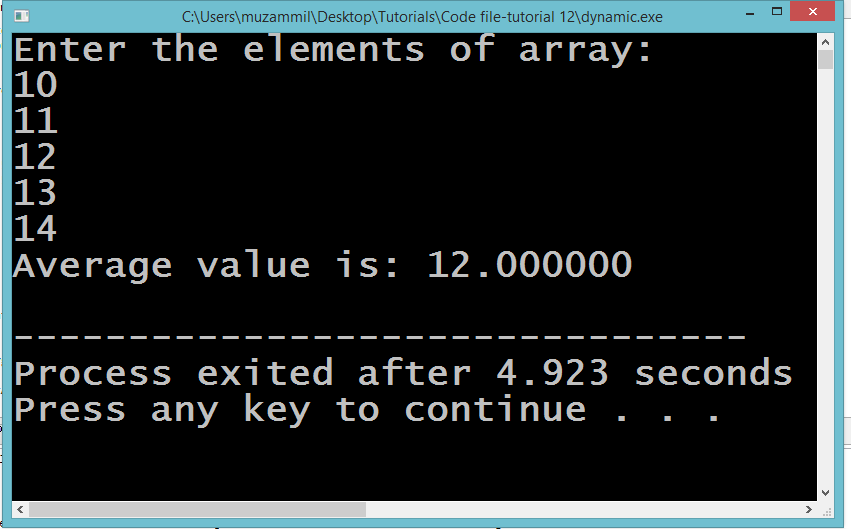# Functions And Pointers in C Language

## Functions and Pointers

In this part you will learn: 1. C syntax 2. Pointers 3. Pointers declaration 4. Function arguments as pointers 5. Showing output In this tutorial we will use pointers with functions. Using functions with pointers is same as using functions with any normal variable. The only difference comes in the parameter list and sometimes in the return type as well when we return a pointer or an address of a variable. Calculating Average Open Dev C++ then File > new > source file and start writing the code below.
`#include<stdio.h>#include<conio.h>`
These two are the most common and basic header files. The first one stdio.h is used to grant us most of the basic functions of C like Input and Output functions. The second one conio.h provides us functions like getch() which is used to pause our screen until we press a button.
`double getAverage(int *arr, int size);int main(){  	int list;   int size=5;   	printf("Enter the elements of array:\n"); 	int i;	for( i=0;i<size;i++)	{		scanf("%d",&list[i]);	}    double avg;    avg = getAverage( list, size ) ;    printf("Average value is: %f\n", avg );    return 0;}`
In this program we will make an array and pass it on to a function which has pointers as arguments. First of all we take input from the user in an integer array. As for the start we have set the array size to 5. Then using a for loop we took input in the array. After that we declared a variable with data type as double. We named the variable with average because our ultimate goal in this program is to calculate the average of all the numbers entered in the array. Then we called our function getAverage() which calculated the average of the numbers entered in the array. We passed on our array containing numbers and the size of the array. Here you have to know one thing that the name of an array is the base address of its first index. So that is why when we pass array to a function that has pointers in its argument we just write the name of the array. If there was any normal variable we would have placed a ampersand sign with the name of the variable because we need to pass the address of the variable to the pointer in the function parameter. Then we printed the average on the screen and the programs ends.
`double getAverage(int *arr, int size){  int    i, sum = 0;         double avg;             for (i = 0; i < size; ++i)  {    sum =sum+ arr[i];  }   avg = (double)sum / size;   return avg;}`
Now see in this function we caught the array in a pointer called arr . In the body of the function we declared some variables to store the average and sum of the numbers of the array. Then we wrote a for loop to add all the numbers of the array in a single variable. After that we divided the sum of the numbers with the size of the array which was basically total number of elements in the array. Then we stored the average in the avg variable. And lastly we returned the average. Execute > compile then Execute > run Output